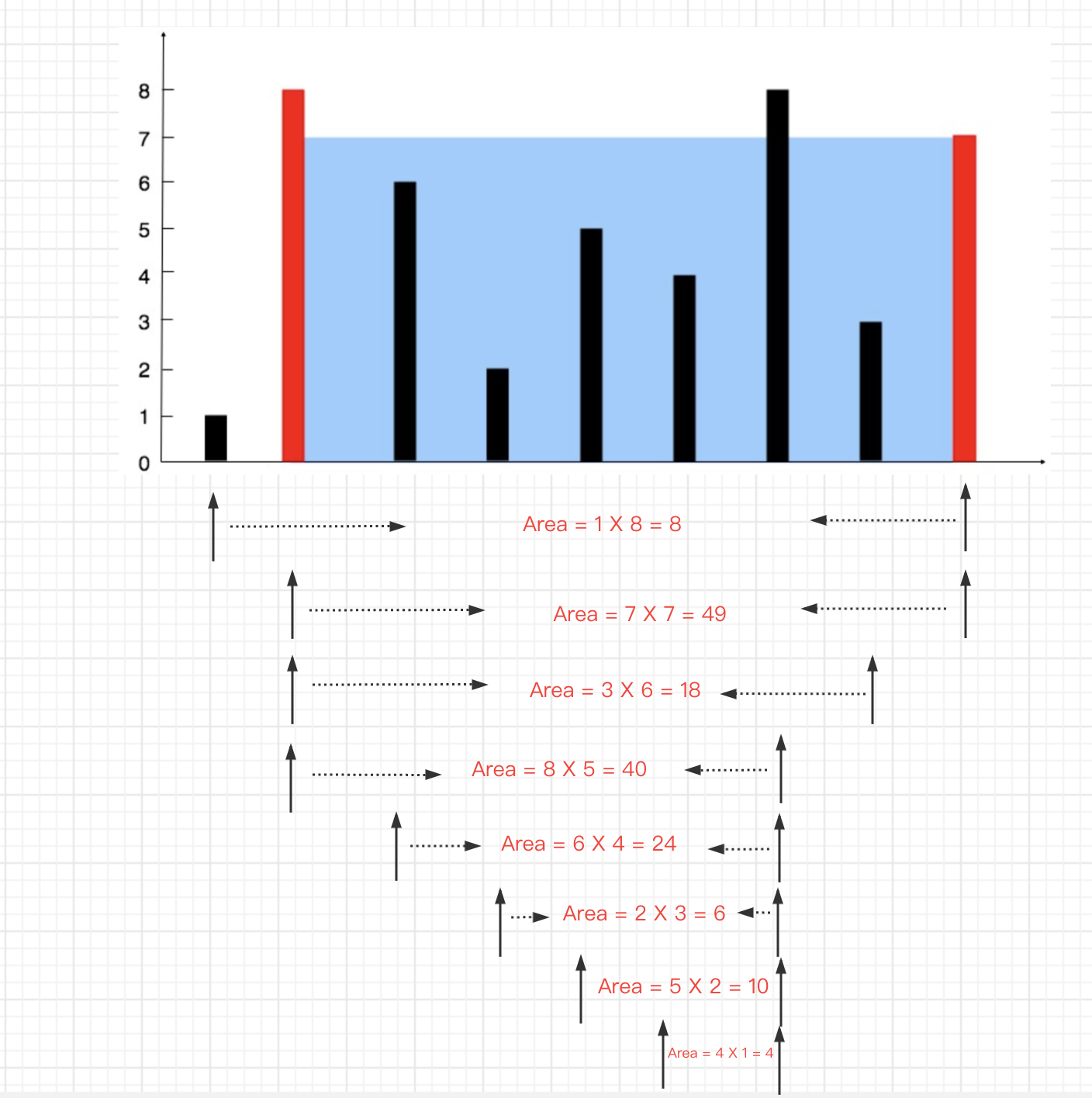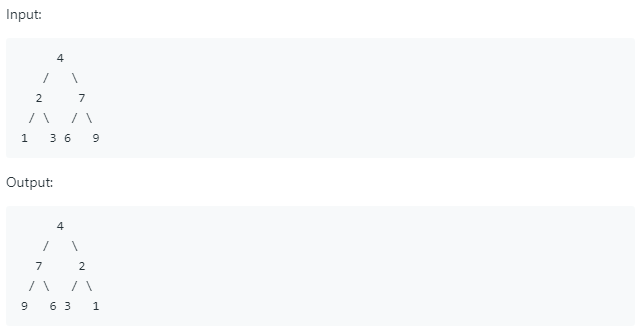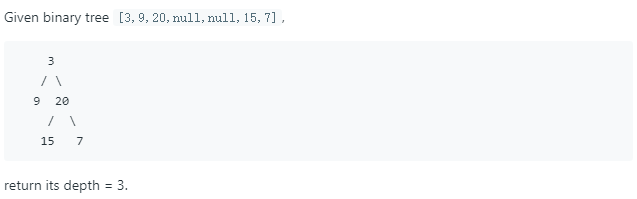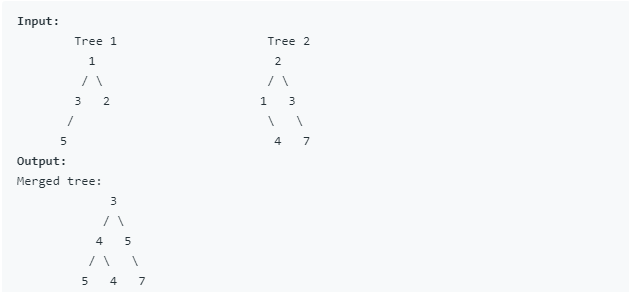• 前言 随着行业不断内卷，手撕leetcode已是进入大厂必备的条件。 笔者网罗了大量BAT等一线互联网大厂笔试面试，对高频题做出了分类总结，题量大约两百，涵盖了剑指与TOP100。...JAVA-高频面试汇总:字
前言
随着行业不断内卷，手撕leetcode已是进入大厂必备的条件。
笔者网罗了大量BAT等一线互联网大厂笔试面试题，对高频题做出了分类总结，题量大约两百题，涵盖了 剑指Offer与 Top100。
每道题的题目都提供了 原题链接 与 最佳题解，算法熟练度极其重要，大家参考我的博客反复练习即可！
专栏目录:
JAVA-高频面试题汇总:动态规划
JAVA-高频面试题汇总:字符串
JAVA-高频面试题汇总:二叉树(上)
JAVA-高频面试题汇总:二叉树(下)
JAVA-高频面试题汇总:回溯
JAVA-高频面试题汇总:链表
JAVA-高频面试题汇总:数组（上）
JAVA-高频面试题汇总:数组（下）
JAVA-高频面试题汇总:堆和栈
LeetCode题型总结还未完结，会持续更新，欢迎关注小编后续的其他专栏！
同时小编在组建实习笔试互助群，下方留下了小编微信二维码，有需要的请联系！
小编微信: Apollo___quan展开全文算法 数据结构
• 两数之和 /* 给定一个整数数组 nums 和一个目标值 target，请你在该数组中找出和为目标值的那两个整数，并返回他们的数组下标。 你可以假设每种输入只会对应一个答案。但是，数组中同一个元素不能使用两遍。...
两数之和
/*
给定一个整数数组 nums 和一个目标值 target，请你在该数组中找出和为目标值的那两个整数，并返回他们的数组下标。
你可以假设每种输入只会对应一个答案。但是，数组中同一个元素不能使用两遍。

给定 nums = [2, 7, 11, 15], target = 9
因为 nums + nums = 2 + 7 = 9
所以返回 [0, 1]
*/
export function twoNumberAdd(nums: number[], target: number) {
// 思路：我们可以在遍历数组的过程中，增加一个Map结构来存储已经遍历过的数字及其对应的索引值。然后每遍历到一个新数字的时候，都回到Map里去查询targetNum与该数的
// 差值是否已经在前面出现过，若出现过则答案已经显现，我们就不在往下走了。
let diffs = {}
for (let i = 0; i < nums.length; i++) {
// 判断当前值与target的差值是否存在
if (diffs[target - nums[i]] !== undefined) {
return [diffs[target - nums[i]], i]
}
// 若没有对应差值，则记录当前值
diffs[nums[i]] = i
}
}


三数之和
/*
三数之和
给你一个包含那个整数的数组nums，判断nums中是否存在三个元素a,b,c使得a+b+c=0，请你找出所有满足条件且重复的三元组。
注意：答案中不可以包含重复的三元组。
示例：
给定数组nums = [-1, 0, 1, 2, -1, -4],
满足条件的三元组集合为
[
[-1, 0, 1],
[-1, -1, 2]
]

思路：
跟两数之和一样，我们把求和的问题变成求差的问题：固定其中一个数，在剩下的数中寻找是否有两个数的和
和这个固定的数相加和是0
这里才用  双指针法  来解决问题，相比三层循环，效率会大大提升。
*/
/*
双指针法的适用范围比较广，一般像求和，比大小都可用它来解决，但是有一个前提：数组必须有序。
*/
/*
因此我们的第一步是给数组进行排序，升序排列
nuns = nums.sort((a, b) => {
return a - b
})
然后对数组进行遍历，每遍历到哪个数字，就固定当前数字。同时左指针指向该数字后面的紧邻的那个数字，
右指针指向数组末尾。然后左右指针分别向中间靠拢
每次指针移动一次位置，就计算一下两个指针指向数字之和加上固定的那个数之后，是否等于0。如果是，
那我们就得到了一个目标组合；否则，分两种情况来看：
1.相加之和大于0，说明右侧的数偏大，右指针左移
2.相加之和小于 0，说明左侧的数偏小了，左指针右移
*/


threeSum(nums: number[]) {
// 用于存放结果的数组
let res: any = []
// 给nums排序
nums = nums.sort((a, b) => {
return a - b
})
// 缓存数组的长度
const len = nums.length
for (let i = 0; i < len - 2; i++) {
// 左指针j
let j = i + 1
// 右指针k
let k = len - 1
// 如果遇到重复的数字 则跳过
if (i > 0 && nums[i] === nums[i - 1]) {
continue
}
while (j < k) {
// 三数之和小于0，左指针前进
if (nums[i] + nums[j] + nums[k] < 0) {
j++
// 处理左指针元素重复的情况
while (j < k && nums[j] === nums[j - 1]) {
j++
}
} else if (nums[i] + nums[j] + nums[k] > 0) {
// 三数之和大于0，右指针后退
k--

// 处理右指针元素重复的情况
while (j < k && nums[k] === nums[k + 1]) {
k--
}
} else {
res.push([nums[i], nums[j], nums[k]])
// 左右指针一起前进
j++
k--
// 若左指针元素重复，跳过
while (j < k && nums[j] === nums[j - 1]) {
j++
}

// 若右指针元素重复，跳过
while (j < k && nums[k] === nums[k + 1]) {
k--
}
}
}
}
// 返回结果数组
return res
}

盛最多水的容器
给你 n 个非负整数 a1，a2，…，an，每个数代表坐标中的一个点  (i, ai) 。在坐标内画 n 条垂直线，垂直线 i  的两个端点分别为  (i, ai) 和 (i, 0)。找出其中的两条线，使得它们与  x  轴共同构成的容器可以容纳最多的水。
说明：你不能倾斜容器，且  n  的值至少为 2。maxArea(heightArr) {
let max = 0
let i = 0
let j = heightArr.length - 1
while (i < j) {
let minHeight = Math.min(heightArr[i], heightArr[j])
let area = (j - i) * minHeight
max = Math.max(max, area)
// 如果左侧小于右侧，则i++，否则j--（这一步其实就是取所有高度中比较高的，我们知道面积等于长*宽）
if (heightArr[i] < heightArr[j]) {
i++
} else {
j--
}
}
}



展开全文• 这个系列是希望帮助大家每天花30分钟的题目了解面试高频题，让大家面试更加游刃有余。 本期我们讲解三道树的简单。树的题目总是会跟递归扯上关系，而递归也是初学者最头疼的题目，因为不好在纸上演算，写着写着就...
我开了一个LeetCode会员，选择了一些高频率题目。这个系列是希望帮助大家每天花30分钟的题目了解面试高频题，让大家面试更加游刃有余。
本期我们讲解三道树的简单题。树的题目总是会跟递归扯上关系，而递归也是初学者最头疼的题目，因为不好在纸上演算，写着写着就搞混了。本期所用的树的数据结构均如下：
class TreeNode {
int val;
TreeNode left;
TreeNode right;
TreeNode(int x) { val = x; }
}

226. 翻转二叉树

题意：翻转一颗二叉树，如下图这是一道很典型的入门递归题，递归函数的一的典型结构就是边界条件+逻辑主体，我们的核心自然是逻辑主体。在本题中，借用一个临时结点temp来交换左右子树的逻辑是很容易想到的。之所以说本题简单，是因为本题即可以从上到下交换也可以从下到上交换，而且逻辑清晰，完整代码如下：
public TreeNode invertTree(TreeNode root) {
if(root==null)
return null;
TreeNode temp;
temp=root.left;
root.left=root.right;
root.right=temp;
invertTree(root.left);
invertTree(root.right);
return root;
}

104. 二叉树的最大深度

题意：给一个二叉树，返回其最大深度，如下图这道题乍一眼看上去，脑海里就跳出一个思路：深度优先遍历每一个结点，记下每一个结点的深度，然后取最大值。于是我们的第一版代码如下：
int maxnum=0;
public int maxDepth(TreeNode root) {
help(root,0);
return maxnum;
}
void help(TreeNode root,int num){
if(root==null){
maxnum=Math.max(num,maxnum);
return;
}
num++;
help(root.left,num);
help(root.right,num);
}

因为在Java里，在函数中改变int的值，在函数外int值不会改变（深层的原因这里不做解释），所以只有在下一次递归的时候num才会+1，我们的代码是有效的。
这道题最后，其实还有一个很惊艳的一行代码搞定问题的答案，代码如下：
int maxDepth(TreeNode root) {return root==null? 0 : Math.max(maxDepth2(root.left), maxDepth2(root.right))+1;}

说是一行代码，其实有点取巧了。这一行代码很简练，但是可读性没有上面那么好，各位同学可以学习学习。
合并二叉树

题意：合并两个二叉树，令null=0，同位置val相加这道题也比较简单，其关键就是两棵树要同时往左或者同时往右递归，代码如下：
public TreeNode mergeTrees(TreeNode t1, TreeNode t2) {
if(t1==null && t2!=null)
return t2;
if(t1==null && t2==null)
return null;
if(t1!=null && t2==null)
return t1;
if(t1!=null && t2!=null){
t1.val=t1.val+t2.val;
t1.left=mergeTrees(t1.left,t2.left);
t1.right=mergeTrees(t1.right,t2.right);
}
return t1;
}

今天我们介绍的几道题都与递归有关，也是最简单的递归。帮助初学的同学从树的角度了解递归，明天我们将介绍树的另外三道简单题，这次偏向于迭代，比如说利用队列、栈等数据结构代替递归操作。
当然，如果有基础的同学已经完全掌握了我们所讲的东西，那么后天，我们就会开始介绍高频题中的中等题，一定会满足大家的。

关注我，更多算法知识点告诉你。展开全文• ## LeetCode高频题

千次阅读 2014-02-03 10:00:54
Data Structure Java source code HashMap/HashSet/Hashtable/LinkedHashMap, ArrayList, Comparator, Iterator -Implement HashMap -Implement peek() function for iterator -LRU cache, implement ...
Fib
strstr
Matrix
-rotate matrix/rectangle
-move a matrix
-spiral matrix I II
Anagrams(find all unique/all non-unique) Hashtable
Two Pointers:
- 2/3/4 sums, 3 sum closet, combination sum
-Sort colors
-Remove duplicates from Array
-Remove duplicates from Sorted Array
Recursion:
-N-Queens I, II
-Generate Parentheses
Bit-Operation
-Single Number
-Single Number II
-O(1) check whether a number is power of 2
-Multiply strings
-Plus one
Pow(x,n)
Sqrt(x)
Valid Number
Data Structure Java source code
-Implement HashMap
-Implement peek() function for iterator
-Singleton
Stack & Queue
-Evaulate reverse polish notation
-Vlaid Parentheses
-Longest vaid parentheses
-Min stack
-Two stack implements a queue
Best Time to buy & sell stocks I, II, III
Maximum subarray
Trailing number of 0s
DP
Unique Path I, II  机器人走迷宫
数学方法
代码实现
如何做LeetCode？
1. Computer：完成题目，写 test cases
2. Paper：重做一遍
3. Computer: 优化代码风格、删除冗余代码
4. Paper: 默写
展开全文• 文章目录位运算常见问题...缺失数字面试16.01.交换数字693.交替位二进制数476.数字的补数371.两整数之和318.最大单词长度乘积 位运算常见问题 191.位1的个数 解法一： 利用x&1返回最低位是否为1，不断的左...算法 动态规划
• } } 二叉树的最大高度 非常经典的递归 最简子问题：找到左孩子和右孩子的高度的最大值再加1 public class Main { public int maxDepth(TreeNode root) { return root == null ? 0 : Math.max(maxDepth(root.left)...数据结构 算法
• class Solution { public ListNode swapPairs(ListNode head) { ListNode node = new ListNode(-1); node.next = head; ListNode pre = node; while (pre.next != null &... ListNode l1 = pre.next, l2 = pre...java...

# leetcode高频题javajava 订阅